By the CIToolkit content team   |   4 minutes read

# Scatter DiagramMany situations require the investigating whether a relationship exists between two or more variables. A line manager, for example, may want to check the relationship between the number of training hours and employee productivity, or if the number of defects is a function of the experience of the person causing it. A call center manager may be interested in studying the relationship between the number of people working on a shift and the average answer time.

A Scatter Diagram is a way of showing whether two variables are correlated or related to each other. It shows patterns in the relationship that cannot be seen by just looking at the data. It is often used as a first step when analyzing and communicating the correlation between pairs of variables, and before conducting advanced statistical techniques (such as regression) to support or reject hypotheses about the data.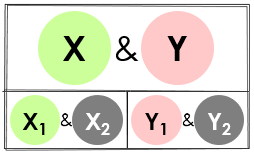A scatter diagram is primarily used to visually investigate the relationship between two variables (often an input and an output variable). This is useful to verify that any change in the input variable will have an effect on the output variable. This information enables you to identify the most significant factors affecting the process and eliminate non-critical factors from consideration.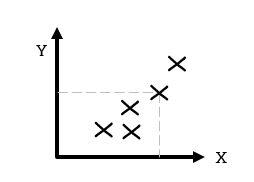A scatter diagram uses a two-axis chart to represent the data. The input variable is plotted along the horizontal axis (x-axis) while the output variable is plotted along the vertical axis (y-axis). You may also study the relationship between two input variables or output variables. In such case, it doesn’t matter which variable goes on which axis. Note that scatter diagrams work with both continuous and count data.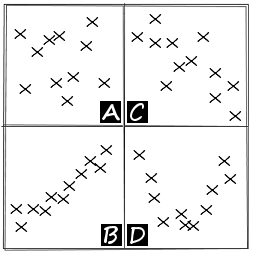Scatter diagrams can indicate several types of correlation:

A) There may be no correlation at all when the data points are scattered randomly without showing any particular pattern.
B) Positive correlation occurs when the values of one variable increase as the values of the other variable increase.
C) Negative correlation occurs when the values of one variable increase as the values of the other variable decrease.
D) Scatter diagrams can also indicate nonlinear relationships between variables.

Be careful before concluding that there is a direct cause-and-effect relationship between the variables. There might be a third factor that is affecting the relationship. No correlation on the other hand does not mean there is no cause-and-effect relationship. There might be a relationship over a wider range of data or a different portion of the range.

## How to Construct a Scatter Diagram

• You must first collect the two paired sets of data.
• Once you have collected enough data points, create a summary table of the data.
• Draw and label the horizontal and vertical axes with variable names and scale values.
• Plot the data pairs on the diagram by placing a dot at the intersection of each data pair.
• Look at how the pattern appears and how the two variables vary together.

## Example

The following is an analysis that shows the relationship between the volume and the diameter of sample trees in a forest (see sample data). The scatter diagram suggests that the two variables are correlated.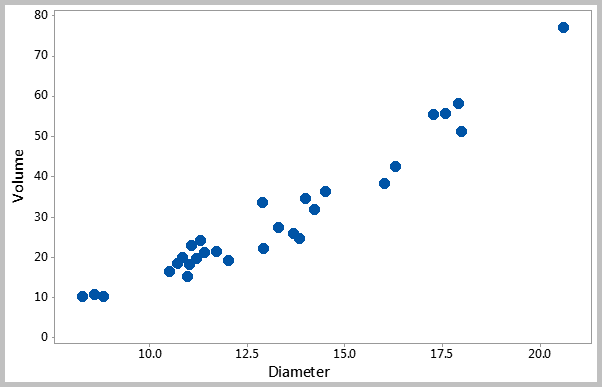The above chart shows the results of a data set that belongs to Minitab Inc.

## Example

The following is an analysis that was conducted for diagnosing the presence of diabetes at a workplace (see sample data). The population was generally young (75.8% were below thirty).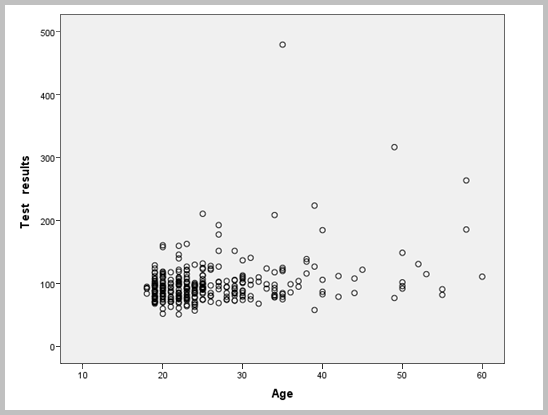The scatter diagram suggests that there is no obvious relationship between age and glucose levels. High glucose levels are found in all ages, and normal glucose levels are found in higher ages.

A Matrix Plot is used to summarize the relationship between pairs of multiple variables in one graph. It produces a scatter diagram for every combination of variables. Potential correlations between pairs of variables can then be identified.

## Example

In the following matrix plot, it appears that there is a positive relationship between the years of experience and salaries. However, the number of publications does not appear to be correlated with the years of experience.

Question: Is there a correlation between the number of publication and salaries?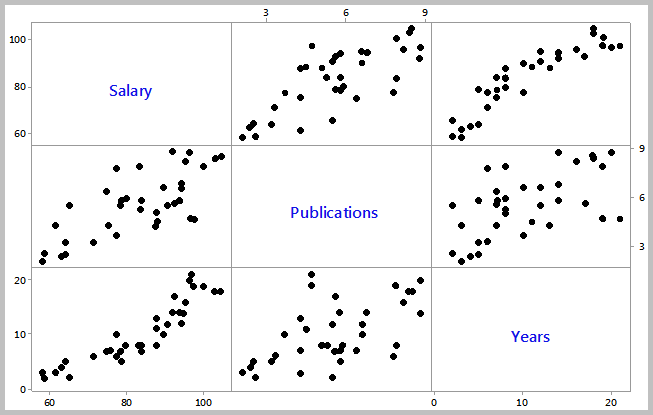The above chart shows the results of a data set that belongs to Minitab Inc.

There are many tools that allow to draw a scatter diagram. One of the simplest ways is to use this scatter diagram template.

## Further Information

• When the relationship is not so clear, Correlation can be used to help determine if a relationship exists between the variables. Regression techniques go a step further by defining the relationship in a mathematical format.
• You can also illustrate a stratification factor in the scatter diagram. For example, the relationship between a process output and a process input for two different settings.

Other Formats

Related Articles

Related Templates